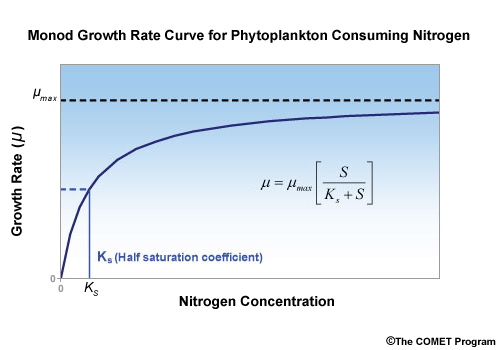## K for NitrogenK is the half-saturation coefficient for Monod formulation uptake. In this case it is phytoplankton consuming the nutrient nitrogen. The half-saturation coefficient determines how well the phytoplankton can grow at low levels of nitrogen. Lower K values means that growth is closer to maximum growth rates for a given level of food.

This plot shows the the uptake rate (μ) of nitrogen by Phytoplankton as a function of the nitrogen concentration (horizontal axis). K is the nitrogen concentration where μ equals one-half of the maximum uptake rate (μmax). Logically, if K is smaller, the curve shifts to the left, meaning that the uptake rate (i.e., growth) is the same for a lower nitrogen concentration. Alternatively, this also means that the uptake rate is greater for the same concentration of nitrogen.